###Author Topic: The incompleteness theorems  (Read 376 times)

#### hubag bohol

• THE SOURCE
••• Posts: 89050
•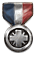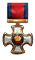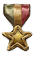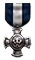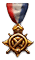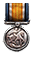• "Better to remain silent and be thought a fool...##### The incompleteness theorems
« on: May 31, 2015, 01:21:14 PM »
GÃ¶del's incompleteness theorems demonstrate that, in mathematics, it is impossible to prove everything.

More specifically, the first incompleteness theorem states that, in any consistent formulation of number theory which is "rich enough" there are statements which cannot be proved or disproved within that formulation. The second incompleteness theorem states that number theory cannot be used to prove its own consistency.

The theorem applies also to any theory which includes number theory, as long as the theory is consistent and as long as the theory is expressed as is usual in mathematics, following rules such as that the axioms and proof procedures are determined from the start and the expressions are of finite length. One major example of such a larger theory in mathematics is set theory, for in set theory one can define numbers and the operations on numbers, and prove the ordinary principles of arithmetic.

GÃ¶del demonstrated this by encoding the liar paradox into number theory itself, creating a well-formed mathematical statement that referred to itself as an unprovable statement. By the assumption of consistency, we know that this statement is true (for, if it were false, then it could be proved, which would be inconsistent). But in virtue of its being true, it cannot be proved (for that is what it says). The final link in the chain of reasoning is the notion of "rich enough," which means that a system contains enough formalism as to be able to describe a statement which refers to itself as an unprovable statement. This is achieved, in part, by showing that (1) statements in arithmetic can be associated with numbers in arithmetic and (2) a proof in arithmetic can be shown to correspond to arithmetical computations on those associated numbers.

The "arithmetic" that the theorem refers to is more than just addition, subtraction, multiplication and division with whole numbers. It also includes statements about "all numbers" or "some numbers," for example, statements about prime numbers; "there is no largest prime number." And there are parts of arithmetic which can be proved to be complete (there is one such part which excludes multiplication), as well as other interesting and complicated areas of mathematics which have been proved to be complete and consistent. So one should be careful when saying that "arithmetic" or "mathematics" is incomplete. Some mathematical theories are complete, for example, Euclidean geometry; its completeness does not contradict GÃ¶del's theorem because geometry does not contain number theory. Also, there is even a proof that arithmetic (in the sense of the incompleteness theorems) is consistent; but that proof relies on methods that go beyond that arithmetic.

In case that you think you can get around this by adding this true (but unprovable) statement as an additional axiom in arithmetic (after all, you know that it is true), what happens is that the proof changes so that it generates yet another statement that refers to its own unprovability from the new, enlarged set of axioms. -- http://rationalwiki.org/

...than to speak out and remove all doubt." - Abraham Lincoln

#### hubag bohol

• THE SOURCE
••• Posts: 89050
•• "Better to remain silent and be thought a fool...##### Re: The incompleteness theorems
« Reply #1 on: May 31, 2015, 07:14:47 PM »
Informally, GÃ¶del's incompleteness theorem states that all consistent axiomatic formulations of number theory include undecidable propositions (Hofstadter 1989). This is sometimes called GÃ¶del's first incompleteness theorem, and answers in the negative Hilbert's problem asking whether mathematics is "complete" (in the sense that every statement in the language of number theory can be either proved or disproved). Formally, GÃ¶del's theorem states, "To every -consistent recursive class  of formulas, there correspond recursive class-signs  such that neither ( Gen ) nor Neg( Gen ) belongs to Flg(), where  is the free variable of " (GÃ¶del 1931).

A statement sometimes known as GÃ¶del's second incompleteness theorem states that if number theory is consistent, then a proof of this fact does not exist using the methods of first-order predicate calculus. Stated more colloquially, any formal system that is interesting enough to formulate its own consistency can prove its own consistency iff it is inconsistent.

Gerhard Gentzen showed that the consistency and completeness of arithmetic can be proved if transfinite induction is used. However, this approach does not allow proof of the consistency of all mathematics. -- http://mathworld.wolfram.com/

###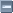Similar Topics###### GÃ¶del's Incompleteness Theorem

Started by hubag bohol on Science and Research

0 Replies
237 Views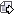August 04, 2013, 03:13:54 PM
by hubag bohol

SMF spam blocked by CleanTalkMobile View